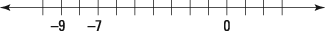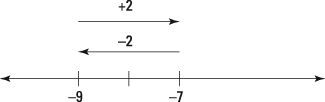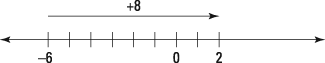##### Common Core Math For Parents For Dummies with Videos OnlineIn Common Core math, seventh grade students add and subtract both positive and negative numbers using the number line. On the number line, numbers have two important meanings:

• A number is a point on the number line.

• A number is a distance on the number line.

What the first one means is that each little tick mark you put on a number line represents a number — 0, 1, 2, and so on: Each of these is matched with a point on the number line.

The number line is a useful tool for adding and subtracting numbers. Many people struggle to explain why –7 – (–9) = 2. But if you think about these numbers on the number line, –7 – (–9) is asking "How far from –9 is –7?" Well, –7 is two spaces to the right of –9 on the number line, so that's the answer, as you see in the figure.The distance between –9 and –7.

The answer to that example (called the difference because it's the answer to a subtraction problem) isn't a point on the number line, though. It's the distance between those two points. Actually, it's a directed distance, which means that keeping track of the direction you go is important when you move between –7 and –9. Moving to the right is positive; moving to the left is negative, as the next figure shows.The directed distances between –9 and –7.

Similarly, you can use number lines to think about addition. For example, 9 + 3 means start at 9, move three spaces to the right. You don't need a number line to know that the sum is 12, but doesn't it help to know that this works out on the number line, too? This idea of start + change = end is more helpful with a problem such as –6 + 8. Start at –6, move 8 spaces to the right, and you end up at 2, as shown in the next figure.Moving 8 spaces to the right of –6.

As another example, –4 + –7 means start at –4 and move 7 spaces to the left. You end at –11, so –4 + –7 = –11.